This video was brought to you by GeneralPAC.com, making power systems Intuitive, Open and Free for Everyone, Everywhere. Consider subscribing and supporting through patreon.com/GeneralPAC. This is a mechanism for you to support us financially so we can continue making high quality power system video tutorials. Our corporate sponsor for this topic is AllumiaX.com from Seattle, Washington. Contact them for industrial and commercial power system studies.

Introduction to current transformers, part three, current transformer ratio.

In part two, we talked about the polarity markings of a current transformer. In part three, we'll talk about current transformer ratio and how to relate primary current to secondary current.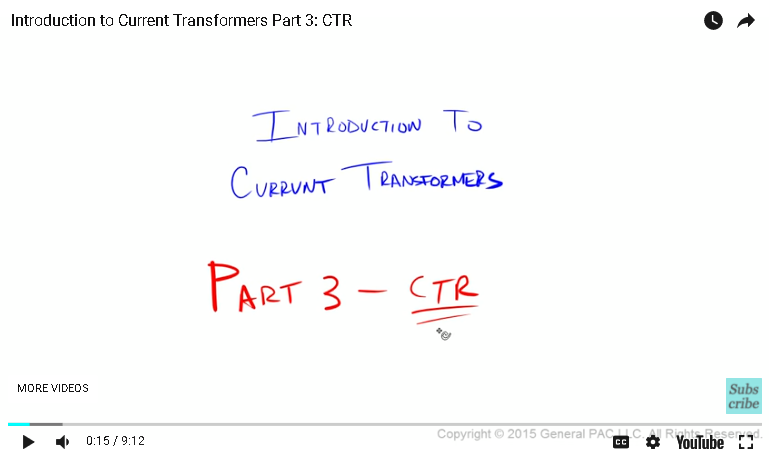Okay, let's first begin by drawing an ideal transformer. An ideal transformer is a transformer that has a primary winding and a voltage across the primary winding that is defined as VP and number of primary turns and current that's going into the primary winding.

Okay, the secondary winding looks something like this. We have the number of secondary turns, which is defined as NS, and then the secondary voltage across the secondary winding, and then the secondary current [inaudible 00:00:49], and then oftentimes we have polarity markings by nice round dots like that. Then we have two lines that are right in the middle of these two windings, which indicate magnetic coupling.

Okay, let's relate primary voltage with secondary voltage. Now, primary voltage is directly proportional to the number of primary terms. Secondary voltage is directly proportional to the number of secondary terms. Primary voltage and the number of primary terms are directly proportional, which means that if we solve for the secondary voltage, it would look something like this.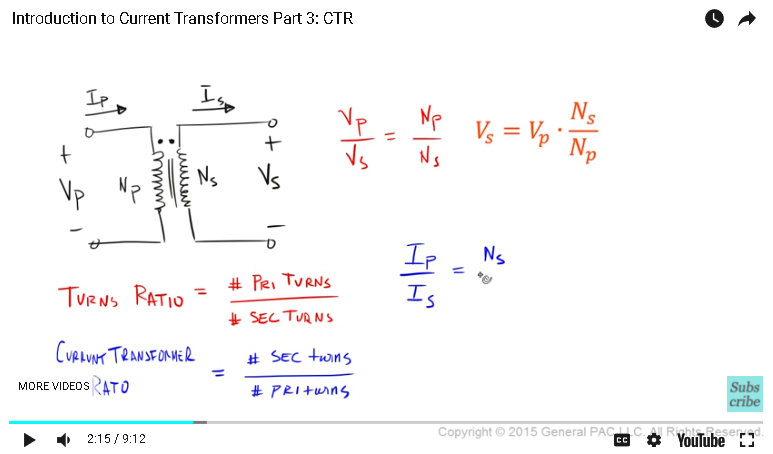Turns ratio in a transformer is defined as the number of primary turns divided by the number of secondary turns. But when we're talking about a current transformer, the current transformer ratio I a little bit different.

The current transformer ratio is equal to the number of secondary turns divided by the number of primary turns. It's essentially flipped. Why is that? Why is it flipped? Let's try to understand this intuitively.

Okay, let's relate primary current to secondary current. Now, if we want to relate primary current to secondary current, here's what we would have to do. Primary current divided by secondary current is direct proportional to the number of secondary turns divided by the number of primary turns.

When we're talking about currents now, look how the primary current, which is this primary current that's going into the primary winding, it's directly proportional to the number of secondary turns, which is this value here. When we're talking about currents, it's flip-flopped. It doesn't hold the same relationship as voltage.

Now, if we solve for the secondary current, it would equal primary current times the number of primary turns divided by the number of second turns. The purpose of a current transformer is to transform primary current, which should be a high value, to secondary current, which should be a low value, but to transform them proportionally.

If we were to design that current transformer, you would want to have a small secondary current. That's the whole purpose of a current transformer. Now, to have a small secondary current means that we either have to have a very small primary turns, or we would have to have a very large secondary turn. Again, secondary current is small when we have a high number of secondary turns or a low number of primary turns.

What does that mean? That means that this picture that I've drawn is technically incorrect because in this picture, the number of primary turns equals the number of secondary turns. This isn't a very good representation of a current transformer.

This is a better representation of a current transformer. We have the primary voltage here, the number of primary turns, primary current here, the number of secondary turns, which is a lot more, and the secondary voltage here, and secondary current falling out like that.

In this example, we have only two turns for the primary winding and a whole lot of turns for the secondary winding, which makes a lot of sense. This is a current transformer. The current transformer ratio, which is this value here, is defined by simply the number of secondary turns, which is this value here, divided by the number of primary turns.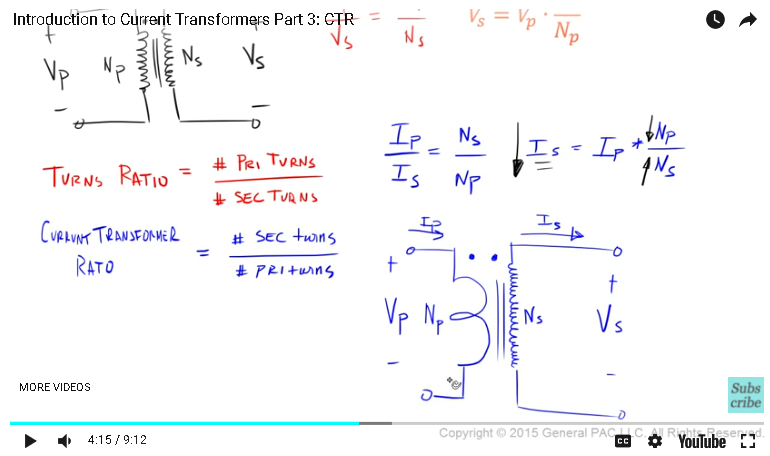Quite often, we see a CTR of, let's say, 600 to five, which means that proportionally, now, we have 600 turns for our secondary winding and only five turns for our primary winding. Now, another way to think about this is essentially 120 to one, which simply means that we have 120 secondary turns and only one primary turn.

Now, a type of current transformer is called a window-type transformer, which we'll draw like this. In the window transformer, there's a big window like that. Quite often, we see that there is a conductor that flows directly through this window-type transformer. The source is on this side and the load is on that side. We know that current moves like that, so let's suppose that this is our primary current.

In terms of secondary windings, let's say we draw our windings like this. Okay, this is the secondary voltage. The number of secondary turns is essentially one, two, three, and four. This is our secondary current that's flowing through. Our secondary terminals are connected to some meter.

Okay, in this particular example, our primary current over our secondary current is equal to the number of secondary turns over the number of primary turns, which means that secondary current is equal to primary current times the number of primary turns divided by the number of secondary turns.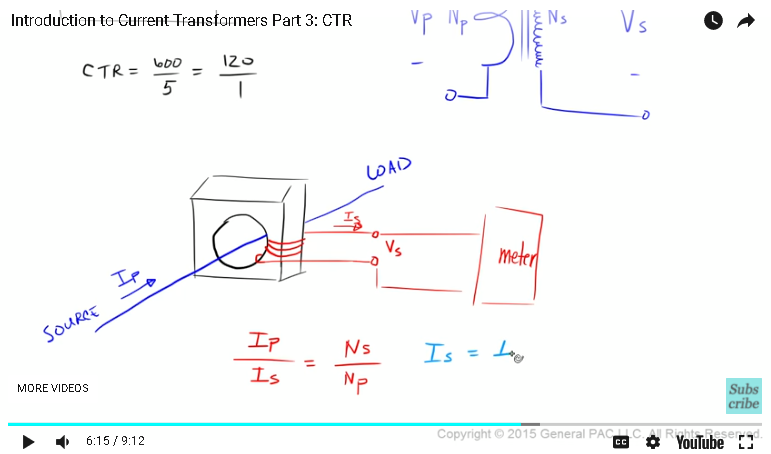Okay, we know that the number of primary turns that we have in our transformer, well, that number is simply one because the conductor goes directly through our transformer. We only have, essentially, one turn. The number of secondary turns is one, two, three, four. The number of secondary turns is four. Now our secondary current is essentially equal to our primary current times one over four, which means that our secondary current is essentially 1/4 of our primary current.

Our CTR is equal to the number of secondary turns divided by the number of primary turns, is equal to four over one. In this particular example, our CTR is essentially four. Very simple, right? Now suppose that we removed our conductor and wrapped it around the CT like this. What did we do here?

We took our conductor and we wrapped it around the CT. Since we wrapped this conductor around the CT like that, now we've actually changed the number of primary turns. Instead of one, it's going to be two. The secondary turns, the number of secondary turns stays the same, which is four. Our equation turns into like this.

This becomes a two. This becomes a two. This becomes two. CTR is essentially the value that is proportional to the number of secondary turns divided by the number of primary turns. CTR is the thing that relates the secondary current to the primary current.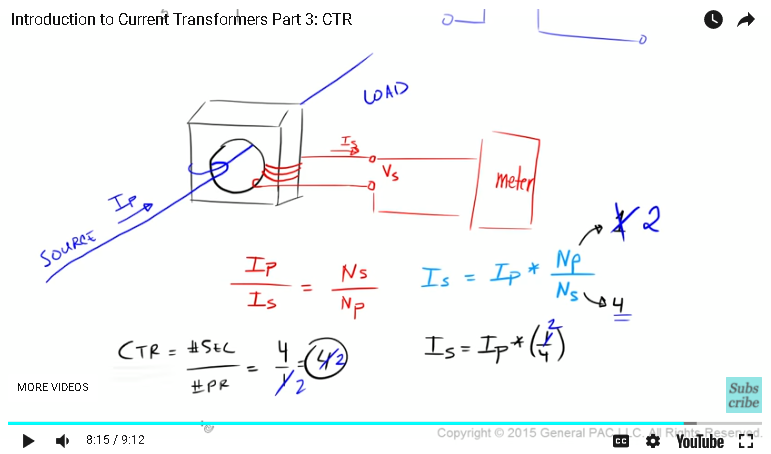In part two, we talked about an ideal transformer. We talked about the primary current. We talked about the number of primary turns, the number of secondary turns, and we talked about this case here. We talked about what turns ratio means in a transformer, and then we talked about current transformer ratio. We also drew our ideal transformer again to better represent what CTs look like, and we did an example where we solved the secondary current based on the number of turns.

In part four, we're going to draw WYE-connected current transformers for three-phase systems. Thank you! This module was brought to you by GeneralPac.com, making power system protection, automation, and controls intuitive.

#### Greetings from the GeneralPAC Team!

We make high-quality Power Systems Video Tutorials on complex topics that are free and open to everyone!  Thank you so much for supporting us through Patreon so we can continue doing good and valuable work.

What is Patreon and why do we use it?

Patreon is a fantastic portal that allows our fans and community to make monthly contribution (like Netflix subscription) so we can continue creating high-quality power systems video tutorials. In return, you get access to incredible perks like voting on future topics, getting your questions answered, access to VIP Q/A webinars with the creators of GeneralPAC, and much more! We THANK YOU for supporting us

Why do we need your support?

An incredible amount of time and effort is needed to develop high-quality video tutorials. Each video (Part 1 for example) takes approximately 10 hours to complete which includes learning the concept ourselves, brainstorming creative ways to teach and explain the concepts, writing the script, audio recording, video recording, and editing. It's no wonder why Hundreds-of-Thousands of people have watched, liked, subscribed, and left positive comments on Youtube channel. Your support truly makes all the difference.